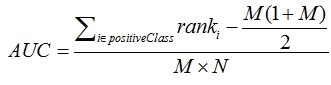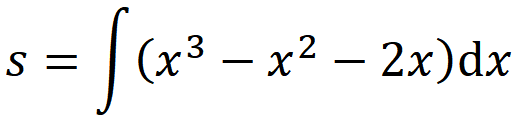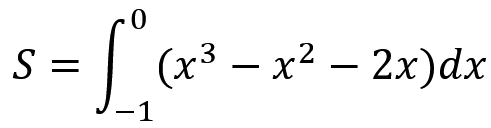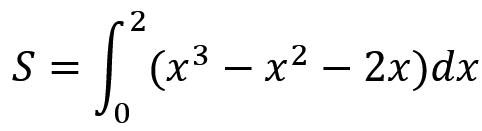• 除了作为 ROC 曲线下面积外，AUC 的解释还鲜为人知： 如果您选择一对随机样本（一个正样本和一个负样本） - AUC 是正样本分数高于负样本分数的概率。 在这里，我们通过计算这些对来计算 AUC。matlab
• matlab开发-曲线下面积曲线下区域的可视化
• 此函数计算封闭曲线 C 所包围的面积，该曲线只能“逆时针”移动，不能交叉循环。 它是 Matlab 的“polyarea”函数的简化版本。 它对多边形的方向和其他能力没有限制。 然而，由于其简单性，它比 matlab 版本快 40%。...matlab
• 滞回环面积求解，实验数据中滞回曲线面积计算，采用MATLAB编写。
• matlab曲线重构代码关于此代码 脚本“ main.m”用于绘制接收器操作特性曲线（ROC曲线）和计算ROC曲线下面积（AUROC） 数字 图1不同筛选方法的ROC曲线和AUROC值我是谁 元重天博士候选医学物理实验室。 -SUMP实验室。 ...
•MATLAB
• IntegralTool 是圣母大学 2011 年Spring工程入门课程... 它利用两个滑块的位置来设置积分极限，计算积分并以数字形式显示，并以图形方式显示曲线下面积。 这包含 IntegralTool.fig、IntegralTool.m 和 calcIntegral.mmatlab
• 在命令行输入%%滞回曲线t0=0;tf=20;x0=[0 0 0][tx]=ode45('Lorenz'[t0 tf]x0);plot(x(:1)x(:3))，可得滞回曲线
• 此函数实现了 Hand 和 Till 对多类分类问题的 ROC 曲线下面积 (AUC) 的泛化（Hand DJ、Till RJ，“A Simple Generalization of the Area Under the ROC Curve for Multiple Class Classification Problems, Machine ...matlab
• 查找正态分布曲线下面积并绘制带有阴影面积的 pdf。 可以采取以下两种路径之一： 1) 欠曲线(pd,'p',a,b) 2) 欠曲线(pd,'v',a,b) pd 是一个分发对象； 使用 path='p' 求样本在正态分布曲线下的比例， 其中 pd 是...matlab
• 此函数计算正态分布曲线下的概率，绘制图形并计算面积。 %正态分布% % 计算正态分布曲线下面积% 从 -ve 无穷大到点 x。 % ％ 输入： % x : 正态分布曲线上的点% mean : 正态分布曲线的平均值% sigma : 正态分布...matlab
• 用于估计接收器操作曲线 (ROC) 和 ROC 曲线下面积 (AUC) 的函数，以及用于估计 AUC 估计的参数和非参数置信区间的各种方法。 还包括针对已知值对 ROC 的估计区域进行简单引导测试的代码。 可用的 CI 估计方法有： ...matlab
• 此函数使用半透明背景绘制一组数据的平均值和标准偏差，填充正负平均误差之间的空间，完全可定制。 输入参数： -数据：数据矩阵，其中的行对应于观测值，而列对应于样本。 - 选项：（可选）包含自定义参数的结构。...matlab
• 此函数计算 ROC（接收者操作符特征）曲线下面积。 该排名指标广泛用于机器学习、统计学、心理物理学等领域。 该函数可以轻松计算和绘制 100 个输入的 AUC 和 ROC 曲线，每个输入的大小为 10^5。matlab
• matlab求曲线的面积 AUROC

万次阅读 2016-01-12 13:53:22
曲线面积的求法： （1）任意曲线的面积求法： （2）ROC曲线的面积求法： function auc=auc(FPR,TPR); % given true positive rate and false positive rate calculates the area under the curve % true positive ...

曲线面积的求法：

（1）任意曲线的面积求法：

>> x=0:pi/100:pi;
>> y=sin(x);
>> trapz(x,y)

ans =

1.9998

（2）ROC曲线的面积求法：

function auc=auc(FPR,TPR)
% given true positive rate and false positive rate calculates the area under the curve
% true positive are on the y-axis and false positives on the x-axis
% sum rectangular area between all points
% example: auc=auc(FPR,TPR);
[x2,inds]=sort(FPR);
x2=[x2,1];  % the trick is in inventing a last point 1,1
y2=TPR(inds);
y2=[y2,1];
xdiff=diff(x2);
%xdiff
xdiff=[x2(1),xdiff];
auc1=sum(y2.*xdiff); % upper point area
auc2=sum([0,y2([1:end-1])].*xdiff); % lower point area
auc=mean([auc1,auc2]);
end

展开全文matlab AUROC
• matlab画ROC曲线

2017-08-31 14:49:34
使用matlab画ROC曲线，% predict - 分类器对测试集的分类结果 % ground_truth - 测试集的正确标签,这里只考虑二分类，即0和1 % auc - 返回ROC曲线曲线下面积
• sklearn.metrics.aucsklearn.metrics.auc(x, y, reorder=False)通用方法，使用梯形规则计算曲线下面积。import numpy as npfrom sklearn import metricsy = np.array([1, 1, 2, 2])pred = np.array([0.1, 0.4, 0.35,...

sklearn.metrics.auc

sklearn.metrics.auc(x, y, reorder=False)

通用方法，使用梯形规则计算曲线下面积。

import numpy as np

from sklearn import metrics

y = np.array([1, 1, 2, 2])

pred = np.array([0.1, 0.4, 0.35, 0.8])

fpr, tpr, thresholds = metrics.roc_curve(y, pred, pos_label=2)

metrics.auc(fpr, tpr)

sklearn.metrics.roc_auc_score

sklearn.metrics.roc_auc_score(y_true, y_score, average='macro', sample_weight=None)

计算预测得分曲线下的面积。

只用在二分类任务或者 label indicator 格式的多分类。

y_true:array, shape = [n_samples] or [n_samples, n_classes]

真实的标签

y_score:array, shape = [n_samples] or [n_samples, n_classes]

预测得分，可以是正类的估计概率、置信值或者分类器方法 “decision_function” 的返回值；

average:string, [None, ‘micro’, ‘macro’ (default), ‘samples’, ‘weighted’]

sample_weight : array-like of shape = [n_samples], optional

import numpy as np

from sklearn.metrics import roc_auc_score

y_true = np.array([0, 0, 1, 1])

y_scores = np.array([0.1, 0.4, 0.35, 0.8])

roc_auc_score(y_true, y_scores)

一个完整的例子

from sklearn import datasets,svm,metrics,model_selection,preprocessing

x=iris.data[iris.target!=0,:2]

x=preprocessing.StandardScaler().fit_transform(x)

y=iris.target[iris.target!=0]

x_train,x_test,y_train,y_test=model_selection.train_test_split(x,y,

test_size=0.1,random_state=25)

clf=svm.SVC(kernel='linear')

clf.fit(x_train,y_train)

metrics.f1_score(y_test,clf.predict(x_test))

0.75

fpr,tpr,thresholds=metrics.roc_curve(y_test,clf.decision_function(x_test),

pos_label=2)

metrics.auc(fpr,tpr)

0.95833333333333337

总结

roc_auc_score 是 预测得分曲线下的 auc，在计算的时候调用了 auc；

def _binary_roc_auc_score(y_true, y_score, sample_weight=None):

if len(np.unique(y_true)) != 2:

raise ValueError("Only one class present in y_true. ROC AUC score "

"is not defined in that case.")

fpr, tpr, tresholds = roc_curve(y_true, y_score,

sample_weight=sample_weight)

return auc(fpr, tpr, reorder=True)

两种方法都可以得到同样的结果。

import numpy as np

from sklearn.metrics import roc_auc_score

y_true = np.array([0, 0, 1, 1])

y_scores = np.array([0.1, 0.4, 0.35, 0.8])

print(roc_auc_score(y_true, y_scores))

0.75

fpr,tpr,thresholds=metrics.roc_curve(y_true,y_scores,pos_label=1)

print(metrics.auc(fpr,tpr))

0.75

需要注意的是，roc_auc_score 中不能设置 pos_label，而在 roc_curve中，pos_label的默认设置如下：

classes = np.unique(y_true)

if (pos_label is None and

not (array_equal(classes, [0, 1]) or

array_equal(classes, [-1, 1]) or

array_equal(classes, ) or

array_equal(classes, [-1]) or

array_equal(classes, ))):

raise ValueError("Data is not binary and pos_label is not specified")

elif pos_label is None:

pos_label = 1.

也就是说，roc_auc_score 中 pos_label 必须满足以上条件，才能直接使用，否则，需要使用 roc_curve 和auc。

import numpy as np

from sklearn import metrics

y = np.array([1, 1, 2, 2])

pred = np.array([0.1, 0.4, 0.35, 0.8])

fpr, tpr, thresholds = metrics.roc_curve(y, pred, pos_label=1)

print(metrics.auc(fpr, tpr))

0.75

print(metrics.roc_auc_score(y,pred))

ValueError: Data is not binary and pos_label is not specified

#pos_label 不符合 roc_curve的默认设置，因此报错，可以修改为

y=np.array([0,0,1,1]) #np.array([-1,-1,1,1])

print(metrics.roc_auc_score(y,pred))

0.75

展开全文• 一、roc曲线 ...1、roc曲线：接收者操作特征(receiveroperating characteristic),roc曲线上每个点反映着对同一信号刺激的感受性。 横轴：负正类率(false postive rate FPR)特异度，划分实

转载至   http://blog.csdn.net/pzy20062141/article/details/48711355

一、roc曲线

横轴：负正类率(false postive rate FPR)特异度，划分实例中所有负例占所有负例的比例；(1-Specificity)

纵轴：真正类率(true postive rate TPR)灵敏度，Sensitivity(正类覆盖率)

2针对一个二分类问题，将实例分成正类(postive)或者负类(negative)。但是实际中分类时，会出现四种情况.

(1)若一个实例是正类并且被预测为正类，即为真正类(True Postive TP)

(2)若一个实例是正类，但是被预测成为负类，即为假负类(False Negative FN)

(3)若一个实例是负类，但是被预测成为正类，即为假正类(False Postive FP)

(4)若一个实例是负类，但是被预测成为负类，即为真负类(True Negative TN)

TP:正确的肯定数目

FN:漏报，没有找到正确匹配的数目

FP:误报，没有的匹配不正确

TN:正确拒绝的非匹配数目

列联表如下，1代表正类，0代表负类：由上表可得出横，纵轴的计算公式：

(1)真正类率(True Postive Rate)TPR: TP/(TP+FN),代表分类器预测的正类中实际正实例占所有正实例的比例。Sensitivity

(2)负正类率(False Postive Rate)FPR: FP/(FP+TN)，代表分类器预测的正类中实际负实例占所有负实例的比例。1-Specificity

(3)真负类率(True Negative Rate)TNR: TN/(FP+TN),代表分类器预测的负类中实际负实例占所有负实例的比例，TNR=1-FPR。Specificity

假设采用逻辑回归分类器，其给出针对每个实例为正类的概率，那么通过设定一个阈值如0.6，概率大于等于0.6的为正类，小于0.6的为负类。对应的就可以算出一组(FPR,TPR),在平面中得到对应坐标点。随着阈值的逐渐减小，越来越多的实例被划分为正类，但是这些正类中同样也掺杂着真正的负实例，即TPR和FPR会同时增大。阈值最大时，对应坐标点为(0,0),阈值最小时，对应坐标点(1,1)。

如下面这幅图，(a)图中实线为ROC曲线，线上每个点对应一个阈值。横轴FPR:1-TNR,1-Specificity，FPR越大，预测正类中实际负类越多。

纵轴TPR：Sensitivity(正类覆盖率),TPR越大，预测正类中实际正类越多。

理想目标：TPR=1，FPR=0,即图中(0,1)点，故ROC曲线越靠拢(0,1)点，越偏离45度对角线越好，Sensitivity、Specificity越大效果越好。

二 如何画roc曲线

假设已经得出一系列样本被划分为正类的概率，然后按照大小排序，下图是一个示例，图中共有20个测试样本，“Class”一栏表示每个测试样本真正的标签（p表示正样本，n表示负样本），“Score”表示每个测试样本属于正样本的概率。接下来，我们从高到低，依次将“Score”值作为阈值threshold，当测试样本属于正样本的概率大于或等于这个threshold时，我们认为它为正样本，否则为负样本。举例来说，对于图中的第4个样本，其“Score”值为0.6，那么样本1，2，3，4都被认为是正样本，因为它们的“Score”值都大于等于0.6，而其他样本则都认为是负样本。每次选取一个不同的threshold，我们就可以得到一组FPR和TPR，即ROC曲线上的一点。这样一来，我们一共得到了20组FPR和TPR的值，将它们画在ROC曲线的结果如下图：AUC(Area under Curve)：Roc曲线下的面积，介于0.1和1之间。Auc作为数值可以直观的评价分类器的好坏，值越大越好。

首先AUC值是一个概率值，当你随机挑选一个正样本以及负样本，当前的分类算法根据计算得到的Score值将这个正样本排在负样本前面的概率就是AUC值，AUC值越大，当前分类算法越有可能将正样本排在负样本前面，从而能够更好地分类。

二、AUC计算

1.  最直观的，根据AUC这个名称，我们知道，计算出ROC曲线下面的面积，就是AUC的值。事实上，这也是在早期 Machine Learning文献中常见的AUC计算方法。由于我们的测试样本是有限的。我们得到的AUC曲线必然是一个阶梯状的。因此，计算的AUC也就是这些阶梯 下面的面积之和。这样，我们先把score排序(假设score越大，此样本属于正类的概率越大)，然后一边扫描就可以得到我们想要的AUC。但是，这么 做有个缺点，就是当多个测试样本的score相等的时候，我们调整一下阈值，得到的不是曲线一个阶梯往上或者往右的延展，而是斜着向上形成一个梯形。此 时，我们就需要计算这个梯形的面积。由此，我们可以看到，用这种方法计算AUC实际上是比较麻烦的。

2. 一个关于AUC的很有趣的性质是，它和Wilcoxon-Mann-Witney Test是等价的。这个等价关系的证明留在下篇帖子中给出。而Wilcoxon-Mann-Witney Test就是测试任意给一个正类样本和一个负类样本，正类样本的score有多大的概率大于负类样本的score。有了这个定义，我们就得到了另外一中计 算AUC的办法：得到这个概率。我们知道，在有限样本中我们常用的得到概率的办法就是通过频率来估计之。这种估计随着样本规模的扩大而逐渐逼近真实值。这 和上面的方法中，样本数越多，计算的AUC越准确类似，也和计算积分的时候，小区间划分的越细，计算的越准确是同样的道理。具体来说就是统计一下所有的 M×N(M为正类样本的数目，N为负类样本的数目)个正负样本对中，有多少个组中的正样本的score大于负样本的score。当二元组中正负样本的 score相等的时候，按照0.5计算。然后除以MN。实现这个方法的复杂度为O(n^2)。n为样本数（即n=M+N）
3.   第三种方法实际上和上述第二种方法是一样的，但是复杂度减小了。它也是首先对score从大到小排序，然后令最大score对应的sample 的rank为n，第二大score对应sample的rank为n-1，以此类推。然后把所有的正类样本的rank相加，再减去M-1种两个正样本组合的情况。得到的就是所有的样本中有多少对正类样本的score大于负类样本的score。然后再除以M×N。即公式解释：

1、为了求的组合中正样本的score值大于负样本，如果所有的正样本score值都是大于负样本的，那么第一位与任意的进行组合score值都要大，我们取它的rank值为n，但是n-1中有M-1是正样例和正样例的组合这种是不在统计范围内的（为计算方便我们取n组，相应的不符合的有M个），所以要减掉，那么同理排在第二位的n-1，会有M-1个是不满足的，依次类推，故得到后面的公式M*(M+1)/2，我们可以验证在正样本score都大于负样本的假设下，AUC的值为1

2、根据上面的解释，不难得出，rank的值代表的是能够产生score前大后小的这样的组合数，但是这里包含了（正，正）的情况，所以要减去这样的组（即排在它后面正例的个数），即可得到上面的公式

另外，特别需要注意的是，再存在score相等的情况时，对相等score的样本，需要 赋予相同的rank(无论这个相等的score是出现在同类样本还是不同类的样本之间，都需要这样处理)。具体操作就是再把所有这些score相等的样本 的rank取平均。然后再使用上述公式。

参考：

展开全文• NURBS曲线、曲面拟合的matlab工具箱，加载到path中，可以随时调用，对机床的刀具补偿曲线拟合具有十分大的帮助
• 从区域的像素表示特别是对于不规则曲线，我们可以准确地找到区域matlab
• Matlab函数用于估计接收器工作曲线（ROC）和ROC曲线下面积（AUC），以及各种方法来估计AUC估计的参数和非参数置信区间。 还包括用于针对已知值对ROC的估计面积进行简单引导测试的代码。 可用的CI估计方法为： ...
• MATLAB计算黎曼积分曲线围成的面积假设一个曲线方程f(x)= x.^3-x.^2-2*x。f(x)与笛卡尔坐标x坐标轴有交点，如图：计算该曲线与x（1设所求面积为S，那么：但是f(x)与x坐标轴相交形成的两块面积，在x区域[-1,0]为正，...
MATLAB计算黎曼积分曲线围成的面积

假设一个曲线方程f(x)= x.^3-x.^2-2*x。
f(x)与笛卡尔坐标x坐标轴有交点，如图：计算该曲线与x（1<= x <=2）围成的面积。显然这是一个黎曼积分计算面积问题。
设所求面积为S，那么：但是f(x)与x坐标轴相交形成的两块面积，在x区域[-1,0]为正，[0,2]为负，因此要对[0,2]区域的面积分开计算，分别为：然后取绝对值相加。

matlab:

syms x f;
f=x.^3-x.^2-2*x;
line=ezplot(f,[-1,2]);
set(line,'Color','r','LineWidth',0.5);

grid on;
hold on;

a=int(f,[-1,0])
b=int(f,[0,2])
a+abs(b)

a =

5/12

b =

-8/3

ans =

37/12
最终结果S=37/12。

展开全文数值分析 matlab 数学 数学建模
• 计算相对于增加的曲线下面积 (AUCi) 和相对于地面的曲线下面积 (AUCg)。 有关计算和解释 AUCi 和 AUCg 的更多信息，请参阅“计算曲线下面积的两个公式表示总激素浓度与时间依赖性变化的度量”（Pruessner 等，2003...matlab
• 源自车辆工程专业，汽车理论课程设计。程序部分参考网络资源，自己是小白，有好多不懂地方，如有错误请大佬指出。
• 可以快速实现从滞回曲线中计算耗能面积，和耗能系数，运行速度快，且稳定。
• ROC 曲线说明了二元分类问题的性能，其中分类基于对不同级别的一组分数进行... ROC 图的曲线下面积 (AUC) 是总体准确度的衡量标准，而 ROCCH 面积是一种上限，它可以通过给定分类器的不同阈值结果的加权组合实现。matlab
• 计算面积并判断闭合曲线的方向。 功能区=变量（C） %VAREA 计算闭合曲线的“符号面积”。 % 注：符号面积在逆时针方向时等于闭合曲线面积，在顺时针方向时等于负面积。 % Negative area 表示大小等于面积但符号为...matlab
• 函数colAUC计算矢量或矢量的ROC曲线下面积（AUC） 矩阵的每一列。 这段代码的主要属性： * 能够处理多维数据。 * 能够处理多类数据集。 * 速度 - 编写此代码是为了计算大量的 AUC 特点，快。 * 提供两种不同的算法 ...matlab
• 求滞回曲线耗能,滞回曲线耗能面积计算,matlab源码
• 背景： 如今，已经提出了各种措施来捕获诊断测试中的歧视，但是接收器工作特性（ROC）曲线（AUC）面积仍然是最受欢迎的度量标准。 研究人员通常会评估新的生物标志物增加 AUC 的能力。 但是，很快变得很明显，...matlab...

matlab曲线下面积matlab 订阅